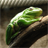PSoC™ 4Level 1Level 1

I am trying to read the voltage of a battery using the SAR ADC but I am not getting the correct values. The ADC outputs 0x7FF0 no matter what the voltage is I am trying to measure. I am using a voltage divider with 357K ohm resistors and an input voltage of 3.8V though this can go as low as 2.5V. The ADC is using an internal Vref of 1.024V. Any help would be appreciated.

1 SolutionLevel 9Level 9

Hi,

If the Vref is 1.024V the input voltage should not exceed that value,

so you need to divide the voltage more ... since you wrote the voltage is 3.8V,

I would try 1/4 dividing.

Meantime, you may not need OpAmp in front of ADC.

motoLevel 9Level 9

Hi,

If the Vref is 1.024V the input voltage should not exceed that value,

so you need to divide the voltage more ... since you wrote the voltage is 3.8V,

I would try 1/4 dividing.

Meantime, you may not need OpAmp in front of ADC.

moto